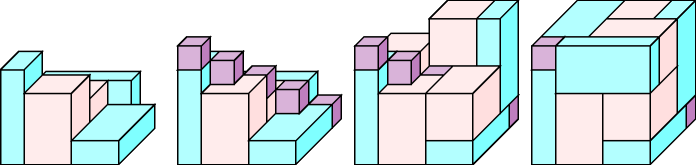### 3.7.65. Conway packing problem

Denotes that a constraint can be used for solving the Conway packing problem, which consists of placing 6 orthotopes of size $4×2×1$, 6 orthotopes of size $3×2×2$ and 5 unit cubes within a $5×5×5$ cube. Figure 3.7.18 shows a solution to the Conway packing problem.

##### Figure 3.7.18. A solution to the Conway packing problem